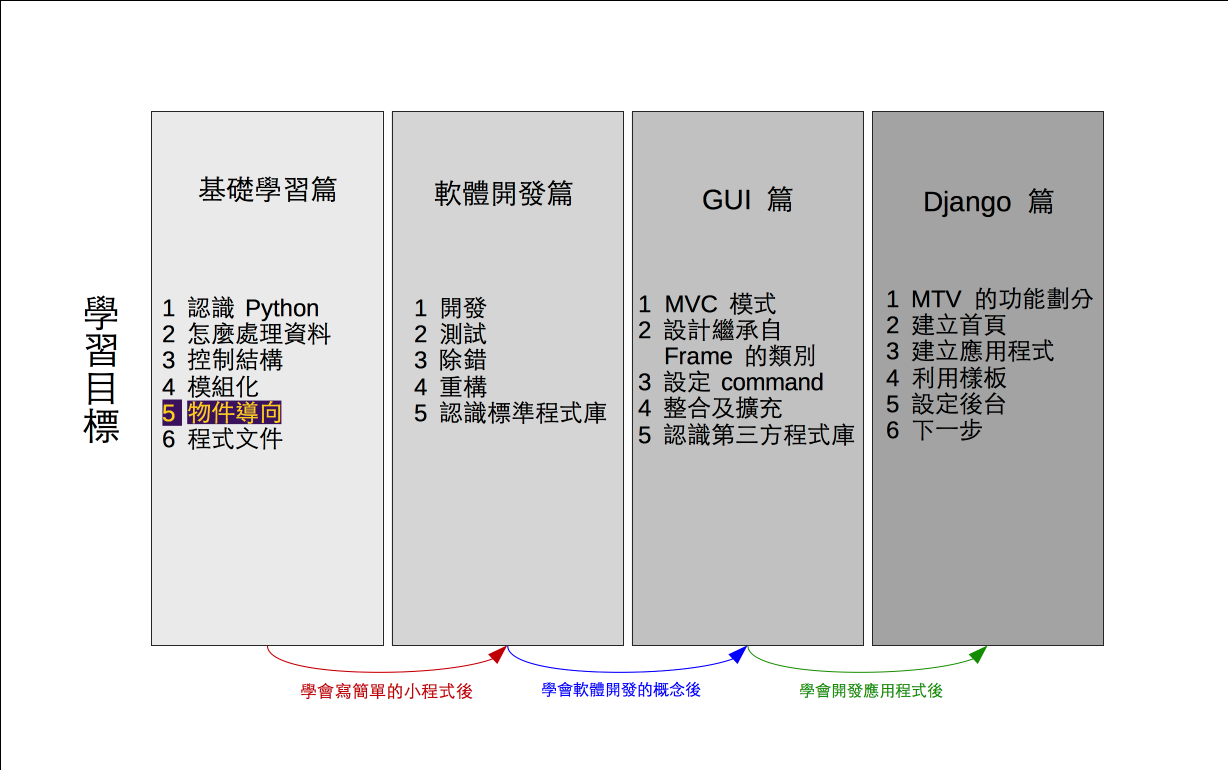# 單元 9 - 類別

～～學習進度表～～class class_name:
class_object_name
def method_name(self):
self.instance

``````class Demo:
def set_att(self, a, b):
self.a = a
self.b = b

def do_something(self):
return self.a + self.b

d = Demo()
d.set_att(11, 22)
print(d.do_something())

#《程式語言教學誌》的範例程式
# http://kaiching.org/
# 檔名：class_demo.py
# 功能：示範 Python 中的類別
# 作者：張凱慶 */``````

class 後面空一格，然後接類別識別字，此例為 Demo ，最後接一個冒號

``class Demo:``

``````class Demo:
def set_att(self, a, b):
self.a = a
self.b = b

def do_something(self):
return self.a + self.b``````

``def set_att(self, a, b):``

set_att() 有兩個參數 ab ，這兩個參數用來設定實體屬性 self.aself.b

``````self.a = a
self.b = b``````

``````d = Demo()
d.set_att(11, 22)
print(d.do_something())``````

 \$ python class_demo.py 33 \$

``````class Demo:
def set_att(self, a=22, b=33):
self.a = a
self.b = b

def do_something(self):
return self.a + self.b

d = Demo()
d.set_att()
print(d.do_something())

#《程式語言教學誌》的範例程式
# http://kaiching.org/
# 檔名：class_demo2.py
# 功能：示範 Python 中的類別
# 作者：張凱慶 */``````

 \$ python class_demo2.py 55 \$

``````class Demo:
a = 11
b = 22

@classmethod
def do_something(cls):
cls.a += 1
cls.b += 1
return cls.a + cls.b

print(Demo.do_something())

#《程式語言教學誌》的範例程式
# http://kaiching.org/
# 檔名：class_demo3.py
# 功能：示範 Python 中的類別
# 作者：張凱慶 */``````

``````class Demo:
a = 11
b = 22``````

``````@classmethod
def do_something(cls):
cls.a += 1
cls.b += 1
return cls.a + cls.b``````

``print(Demo.do_something())``

 \$ python class_demo3.py 35 \$

Python 的屬性都預設為公開，就是可以在類別以外的地方存取，接下來我們介紹如何限制屬性只能在類別中存取，也就是把屬性封裝 (encapsulate) 在類別中。

1. 類別是物件設計的藍圖，每一個類別都可定義屬性及方法。
2. 方法如同函數，專屬於類別。實體屬性屬於由類別建立的物件，類別屬性則屬於類別，須由類別名稱調用。
3. self 為預設的的參數名稱，表示物件實體。
4. __init__() 是類別定義中特殊的方法，用來建立物件。

1. 方法跟函數有什麼異同？
2. 為什麼要有類別屬性？類別屬性跟實體屬性有什麼不同？
3. 如果不寫 self ，那可以用其他的字代替嗎？

1. 寫一個程式 exercise0901.py ，裡頭設計一個類別 IntegerDemo ， 利用 set_value() 方法設定實體屬性 value ，另外定義 add() 方法， add() 需要一個參數 p ，其內容為 value 加上 p ，執行部分先將 value 設定為 25 ，然後再呼叫 add() 並以 24 當參數。
2. 承上題，另寫一個程式 exercise0902.py ，改成由使用者輸入兩個整數。
3. 承上題，另寫一個程式 exercise0903.py ，加入 subtract() 方法， subtract() 是將 value 減去參數值，最後結果儲存在 value
4. 承上題，另寫一個程式 exercise0904.py ，繼續擴充 IntegerDemo ，加入乘法的方法。
5. 承上題，另寫一個程式 exercise0905.py ，繼續擴充 IntegerDemo ，加入除法的方法。
6. 寫一個程式 exercise0906.py ，仿照 IntegerDemo ，將方法改成計算階層值，結果儲存在 value
7. 寫一個程式 exercise0907.py ，仿照 IntegerDemo ，將方法改成計算費氏數列，結果儲存在 value